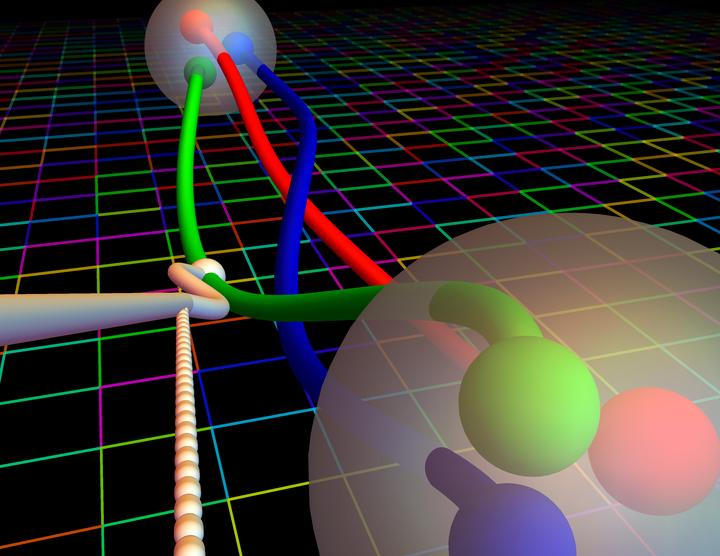# A per-cent-level determination of the nucleon axial coupling from quantum chromodynamicsCredits: Dr. Evan Berkowitz.

### Abstract

The axial coupling of the nucleon, $g_A$, is the strength of its coupling to the $\textit{weak}$ axial current of the Standard Model of particle physics, in much the same way as the electric charge is the strength of the coupling to the electromagnetic current. This axial coupling dictates the rate at which neutrons decay to protons, the strength of the attractive long-range force between nucleons and other features of nuclear physics. Precision tests of the Standard Model in nuclear environments require a quantitative understanding of nuclear physics rooted in Quantum Chromodynamics, a pillar of the Standard Model. The prominence of $g_A$ makes it a benchmark quantity to determine theoretically - a difficult task because quantum chromodynamics is non-perturbative, precluding known analytical methods. Lattice Quantum Chromodynamics provides a rigorous, non-perturbative definition of quantum chromodynamics that can be implemented numerically. It has been estimated that a precision of two percent would be possible by 2020 if two challenges are overcome: contamination of $g_A$ from excited states must be controlled in the calculations and statistical precision must be improved markedly. Here we report a calculation of $g_A^{QCD} = 1.271\pm0.013$, using an unconventional method inspired by the Feynman-Hellmann theorem that overcomes these challenges.

Type
Publication
Nature 558, 91–94 (2018)

Institutional press releases and news articles:##### Enrico Rinaldi
###### Research Scientist

My research interests include artificial intelligence and quantum computing applied to particle physics and quantum many-body systems.